NCERT Solutions (Ex - 13.2) - Direct and Inverse Proportions

# NCERT Solutions (Ex - 13.2) - Direct and Inverse Proportions Notes - Class 8

## Document Description: NCERT Solutions (Ex - 13.2) - Direct and Inverse Proportions for Class 8 2022 is part of Class 8 preparation. The notes and questions for NCERT Solutions (Ex - 13.2) - Direct and Inverse Proportions have been prepared according to the Class 8 exam syllabus. Information about NCERT Solutions (Ex - 13.2) - Direct and Inverse Proportions covers topics like and NCERT Solutions (Ex - 13.2) - Direct and Inverse Proportions Example, for Class 8 2022 Exam. Find important definitions, questions, notes, meanings, examples, exercises and tests below for NCERT Solutions (Ex - 13.2) - Direct and Inverse Proportions.

Introduction of NCERT Solutions (Ex - 13.2) - Direct and Inverse Proportions in English is available as part of our Class 8 preparation & NCERT Solutions (Ex - 13.2) - Direct and Inverse Proportions in Hindi for Class 8 courses. Download more important topics, notes, lectures and mock test series for Class 8 Exam by signing up for free. Class 8: NCERT Solutions (Ex - 13.2) - Direct and Inverse Proportions Notes - Class 8
 1 Crore+ students have signed up on EduRev. Have you?

Exercise 13.2

Question 1:

Which of the following are in inverse proportion:

(i) The number of workers on a job and the time to complete the job.

(ii) The time taken for a journey and the distance travelled in a uniform speed.

(iii) Area of cultivated land and the crop harvested.

(iv) The time taken for a fixed journey and the speed of the vehicle.

(v) The population of a country and the area of land per person.

(i) The number of workers and the time to complete the job is in inverse proportion because less workers will take more time to complete a work and more workers will take less time to complete the same work.

(ii) Time and distance covered in direct proportion.

(iii) It is a direct proportion because more are of cultivated land will yield more crops.

(iv) Time and speed are inverse proportion because if time is less, speed is more.

(v) It is a inverse proportion. If the population of a country increases, the area of land per person decreases.

Question 2:

In a Television game show, the prize money of Rs. 1,00,000 is to be divided equally amongst the winners. Complete the following table and find whether the prize money given to an individual winner is directly or inversely proportional to the number of winners:

 No. of winners 1 2 4 5 8 10 20 Prize for each winner (in rs.) 1,00,000 50,000 .... ..... .... ..... ....

Here number of winners and prize money are in inverse proportion because winners are increasing, prize money is decreasing.

When the number of winners are 4, each winner will get = 100000 /4 = Rs. 25,000

When the number of winners are 5, each winner will get = 100000/5  = Rs. 20,000

When the number of winners are 8, each winner will get = 100000 / 8 Rs. 12,500

When the number of winners are 10, each winner will get 100000/10 = Rs. 10,000

When the number of winners are 20, each winner will get 100000 / 20 = Rs. 5,000

Question 3:

Rehman is making a wheel using spokes. He wants to fix equal spokes in such a way that the angles between any pair of consecutive spokes are equal. Help him by completing the following table:

 No. of spokes 4 6 8 10 12 Angle between a pair of consecutive spokes 90o 60o — — —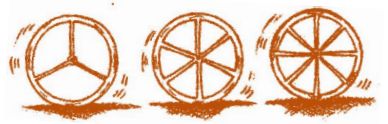(i) Are the number of spokes and the angles formed between the pairs of consecutive spokes in inverse proportion?

(ii) Calculate the angle between a pair of consecutive spokes on a wheel with 15 spokes.

(iii) How many spokes would be needed, if the angle between a pair of consecutive spokes is 40o?

Here the number of spokes are increasing and the angle between a pair of consecutive spokes is decreasing. So, it is a inverse proportion and angle at the centre of a circle is 360 . When the number of spokes is 8, then angle between a pair of consecutive spokes = 360o

When the number of spokes is 8, then angle between a pair of consecutive spokes = 360/8 = 450

When the number of spokes is 10, then angle between a pair of consecutive spokes= 3600/10 = 360

When the number of spokes is 12, then angle between a pair of consecutive spokes = 3600/12 = 300

 No. of spokes 4 6 8 10 12 Angle between a pair of consecutive spokes 900 600 45° 360 300

(I) Yes, the number of spokes and the angles formed between a pair of consecutive spokes is in inverse proportion.

(ii) When the number of spokes is 15, then angle between a pair of consecutive spokes =360/15 =  240 .

(iii) The number of spokes would be needed = 360/40 = 9

Question 4:

If a box of sweets is divided among 24 children, they will get 5 sweets each. How many would each get, if the number of the children is reduced by 4?

Each child gets = 5 sweets

24 children will get 24x5 = 120 sweets

Total number of sweets = 120

If the number of children is reduced by 4, then children left =24-4 = 20

Now each child will get sweets = 120/20 = 6 sweets

Question 5:

A farmer has enough food to feed 20 animals in his cattle for 6 days. How long would the food last if there were 10 more animals in his cattle?

Let the number of days be x.

Total number of animals = 20 + 10 = 30

 Animals 20 30 Days 6 X

Here, the number of animals and the number of days are in inverse proportion.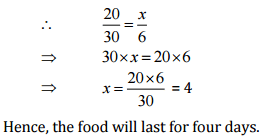Question 6:

A contractor estimates that 3 persons could rewire Jasminder’s house in 4 days. If, he uses 4 persons instead of three, how long should they take to complete the job?

Let time taken to complete the job be x

 Persons 3 4 Days 4 X

Here the number of persons and the number of days are in inverse proportion.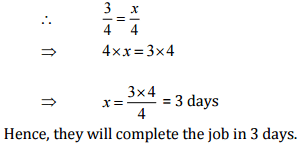Question 7:

A batch of bottles was packed in 25 boxes with 12 bottles in each box. If the same batch is packed using 20 bottles in each box, how many boxes would be filled?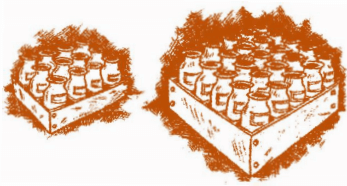Let the number of boxes be x.

 Mo. of bottles in each box 12 20 Boxes 25 x

Here the number of bottles and the number of boxes are in inverse proportion.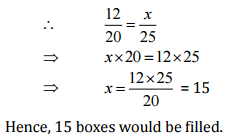Question 8:

A factory requires 42 machines to produce a given number of articles in 63 days. How many machines would be required to produce the same number of articles in 54 days?

Let the number of machines required be x.

 Days 63 54 Machines 42 x

Here, the number of machines and the number of days are in inverse proportion.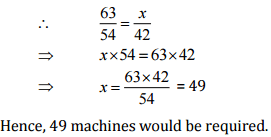Question 9:

A car takes 2 hours to reach a destination by travelling at the speed of 60 km/hr. How long will it take when the car travels at the speed of 80 km/hr?

Let the number of hours be x.

 Speed (in km/hr) 60 80 Time (in hours) 2 X

Here, the speed of car and time are in inverse proportion.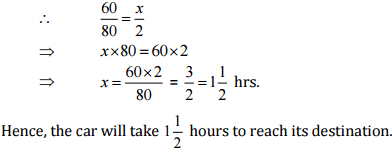Question 10:

Two persons could fit new windows in a house in 3 days.

(i) One of the persons fell ill before the work started. How long would the job take now?

(ii) How many persons would be needed to fit the windows in one day?

(i) Let the number of days be x.

 Persons 2 1 Days 3 x

Here, the number of persons and the number of days are in inverse proportion.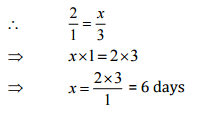(ii) Let the number of persons be x.

 Persons 2 x Days 3 1

Here, the number of persons and the number of days are in inverse proportion.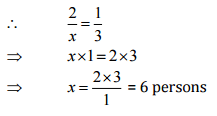Question 11:

A school has 8 periods a day each of 45 minutes duration. How long would each period be, if the school has 9 periods a day, assuming the number of school hours to be the same?

Let the duration of each period be x.

 Period 8 9 Duration of period (in minutes) 45 X

Here the number of periods and the duration of periods are in inverse proportion.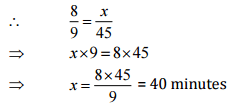Hence, the duration of each period would be 40 mints

The document NCERT Solutions (Ex - 13.2) - Direct and Inverse Proportions Notes - Class 8 is a part of Class 8 category.
All you need of Class 8 at this link: Class 8
 Use Code STAYHOME200 and get INR 200 additional OFF

Track your progress, build streaks, highlight & save important lessons and more!

,

,

,

,

,

,

,

,

,

,

,

,

,

,

,

,

,

,

,

,

,

;